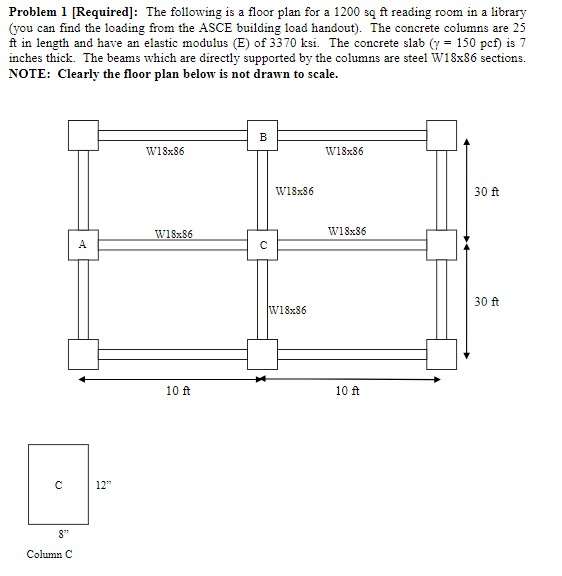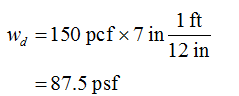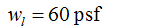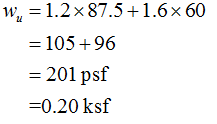# Problem 1 [Required]: The following is a floor plan for a 1200 są ft reading room in a library(you can find the loading from the ASCE building load handout). The concrete columns are 25ft in length and have an elastic modulus (E) of 3370 ksi. The concrete slab (y = 150 pcf) is 7inches thick. The beams which are directly supported by the columns are steel W18x86 sections.NOTE: Clearly the floor plan below is not drawn to scale.w18x86W18x86W18x8630 ftW18x86W18x8630 ftW18x8610 ft10 ft12"8"Column C

Question
6 views
The following is a floor plan for a 1200 sq ft reading room in a library (you can find the loading from the ASCE building load handout). The concrete columns are 25 ft in length and have an elastic modulus (E) of 3370 ksi. The concrete slab (γ = 150 pcf) is 7 inches thick. The beams which are directly supported by the columns are steel W18x86 sections. NOTE: Clearly the floor plan below is not drawn to scale.

(d) Calculate the loading demand on column C (neglect the self-weight of the columns) [answer in lbs or kips] NOTE: You can ignorethe reduced live load factor given in equation 1-1 ofthe textbook even though this column would satisfy KLLAT> 400 ft^2.

(e) Will column C buckle if the ends are pinned(k = 1.0)?

(f) If the deflection limits set forth by the ASCE building code are [in]less than L/360, does beam B-C pass? (note: Esteel= 29,000 ksi, Ix,beam= 1530 in^4)help_outlineImage TranscriptioncloseProblem 1 [Required]: The following is a floor plan for a 1200 są ft reading room in a library (you can find the loading from the ASCE building load handout). The concrete columns are 25 ft in length and have an elastic modulus (E) of 3370 ksi. The concrete slab (y = 150 pcf) is 7 inches thick. The beams which are directly supported by the columns are steel W18x86 sections. NOTE: Clearly the floor plan below is not drawn to scale. w18x86 W18x86 W18x86 30 ft W18x86 W18x86 30 ft W18x86 10 ft 10 ft 12" 8" Column C fullscreen
check_circle

Step 1

d)

Self-weight of the slab is calculated as,Step 2

The ultimate load is calculated as,Step 3

The area of tributary for column is calculated as,

...

### Want to see the full answer?

See Solution

#### Want to see this answer and more?

Solutions are written by subject experts who are available 24/7. Questions are typically answered within 1 hour.*

See Solution
*Response times may vary by subject and question.
Tagged in